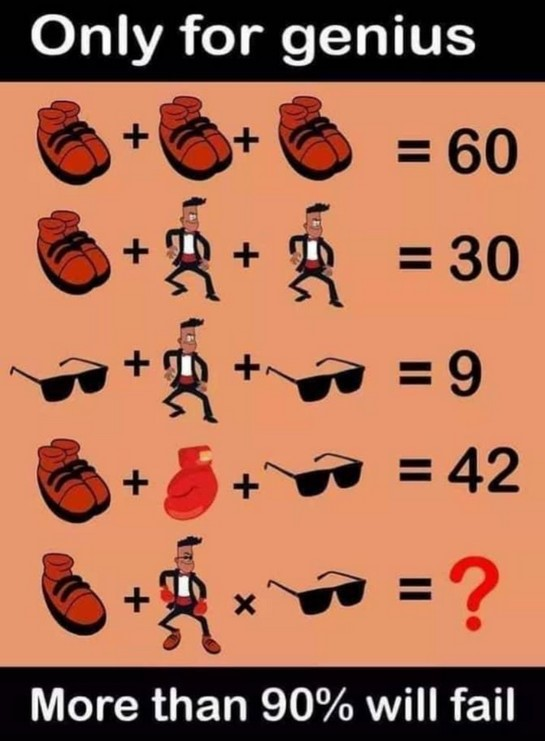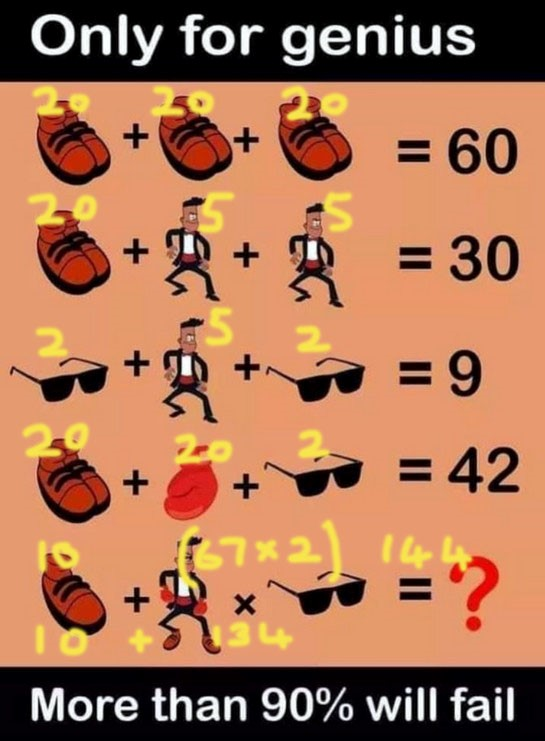## Recommended Posts

The Shoe, Goggles, Man and Boxing Gloves Puzzle

Check out this new genius puzzle on the internet getting viral for a reason.

The Shoe, Goggles, Man and Boxing gloves Puzzle. Only for genius puzzle, more than 90% will fail to solve this genius math puzzle.

Can you solve this viral logical math puzzle? Check out the image and share your equation or answer in the comment section below. Best of luck!Spoiler1 shoe (10) + Man w/2 gloves and glasses and 2 shoes (5+20+20+2+20) x glasses (2)

(10) + (20+20+10+10+5+2)*2

(10) + (67) * 2 = (10) + 134 = 144

Let us first establish the values of each item given in the puzzle. As per the first equation, the addition of three pairs of shoes is 60. This means that one pair of shoes is 20.

The next equation shows that one pair of shoes with two men is 30. While a pair of shoes value 20, this means that one man values for 5.

Keeping in mind the fact that the man values 5, and the equation adds on to 9, the two sunglasses are worth 4. This means that one sunglasses is of the value 2.

A pair of shoes, with one glove and one sunglasses, equal to 42. While the pair of shoe values 20, and one pair of sunglasses values 2. That means, one glove is of 20.

Now comes the real trick. One pair of shoes values 20, which mean a single shoe is of 10. The man can be seen wearing a pair of shoes, a pair of gloves as well as sunglasses. The value of man will be added with the rest to make one whole value.

Man- 5, Gloves- 40 (One glove valued 20 as established), Pair of shoes-20 and Sunglasses-2. All of this equals 67. Looking at the law of BODMAS in math, multiplication of the man along with the glasses in the equation will be done first.

Thus, 67 multiplied by 2 is 134.

Adding the value of 10 of the single shoe, the final answer is 144.